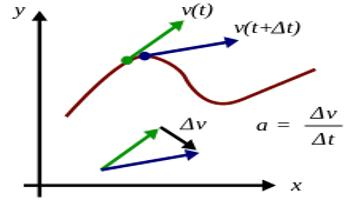# What is Acceleration?

If the magnitude or the direction or both of the velocity changes with respect to time, the particle is said to be under acceleration.

Acceleration of a particle is defined as the rate of change of velocity. Acceleration is a vector quantity.Acceleration = change in velocity / time taken

If u is the initial velocity and v, the final velocity of the particle after a time t. then the acceleration.

a = (v – u)/t

Its unit is ms-2 and its dimensional formula is LT-2.

The instantaneous acceleration is, a = dv/dt =  d/dt (ds/dt) = (d2s/dt2).